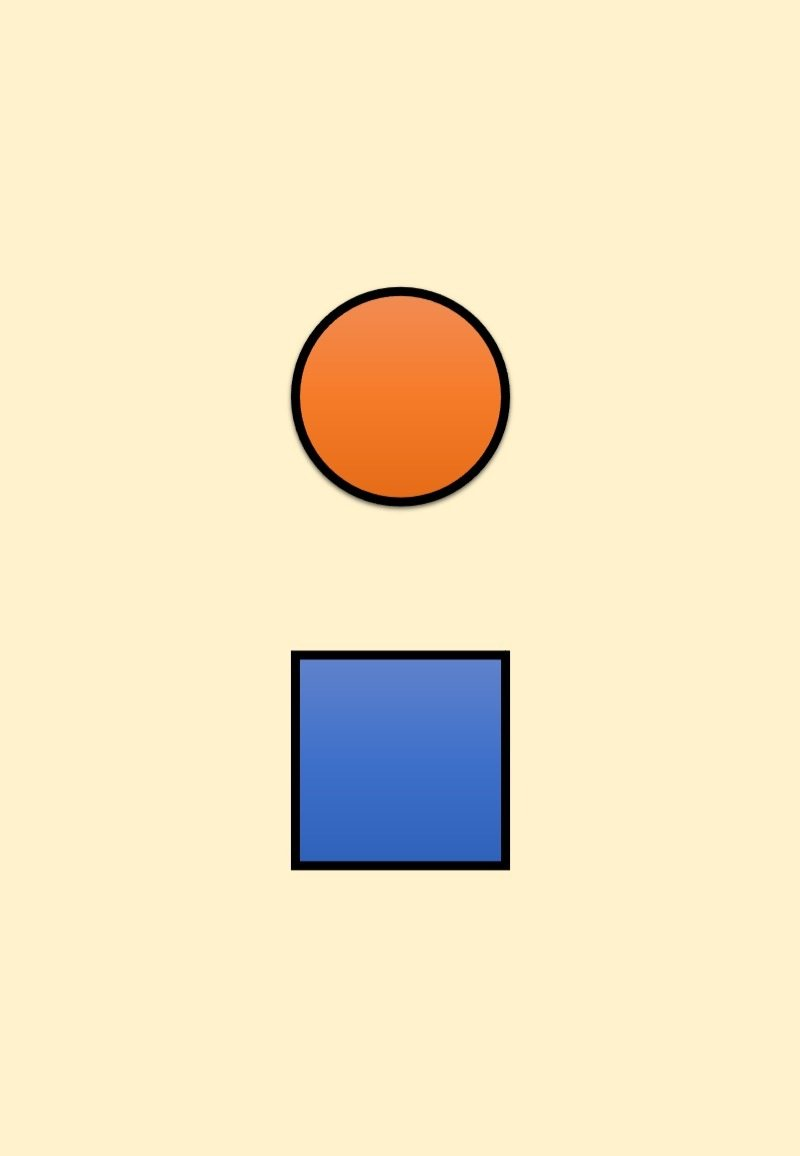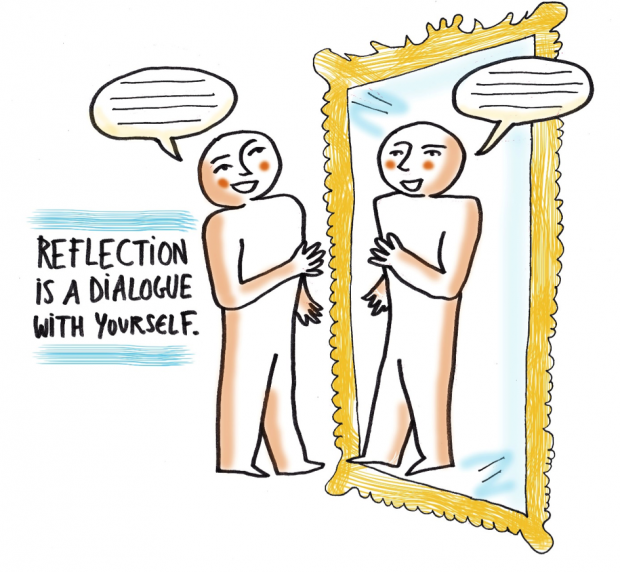# Binary Numbers

based on resources from Code.org and geeks

## What can we communicate using only two symbols? Is there a limit?

In the last Unit, you created your own number system using circles and squares.

What can we communicate using only two symbols? Is there a limit?## Binary Numbers## Flippy Do

Students can complete the tasks individually using the digital manipulatives.

Using flippy Do represent these decimal numbers in binary

• 7
• 20

Using flippy do represent the following two binary digits in decimal format

• 0001 0010
• 0001 1111

Each task can be worked on a different slide

You will find your digital manipulative once you open the assignment in Google Classroom.## Reflection

It’s important to know the differences between binary and decimal number systems.

As a review, the decimal number system is base-10. There are ten different symbols used to represent numbers (0-9). The binary numbers system is base-2. There are two different symbols used to represent numbers (0-1).

Using our Flippy Do, we can convert between Binary and Decimal number systems.

While it is easier for humans to use the decimal number system in our everyday lives, we will see later in this course how electrical signals inside computers can be best represented by using the the binary number system.

Think

• How many bits would be needed to count all of the students in our class?
• Each time we add another bit, what happens to the amount of numbers we can make?
• What are the similarities and differences between the binary and decimal systems?